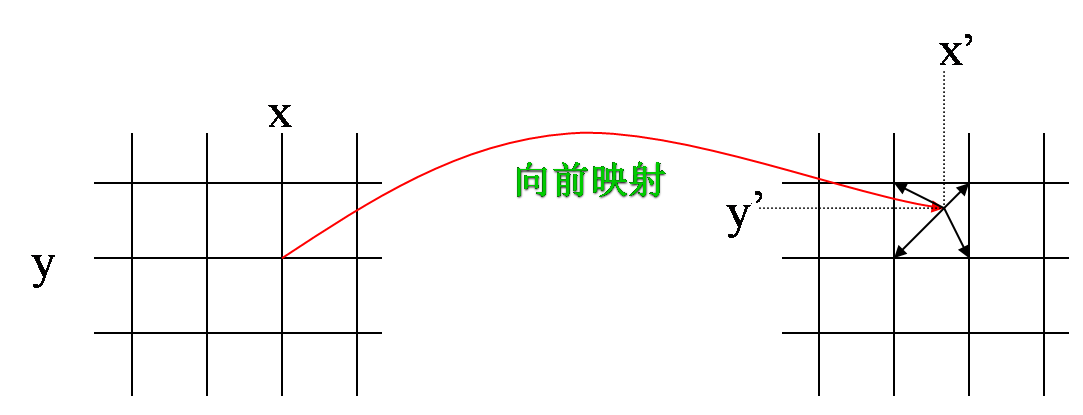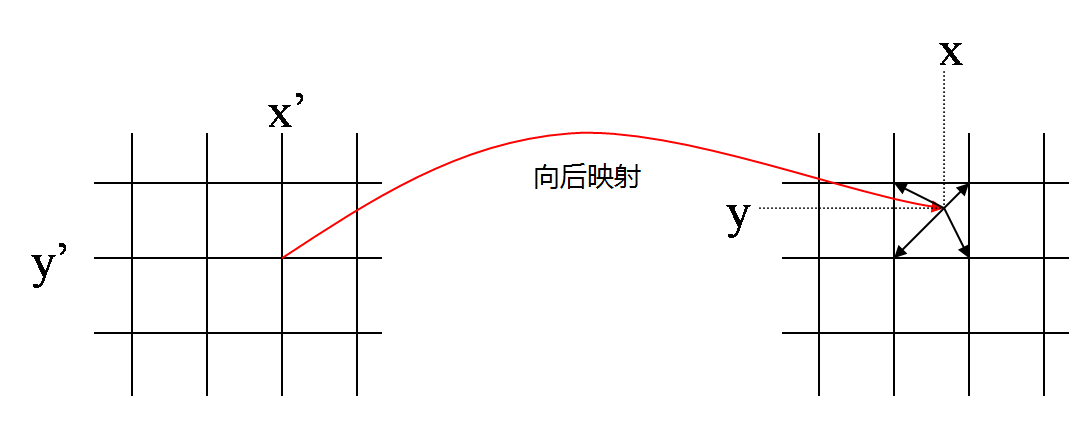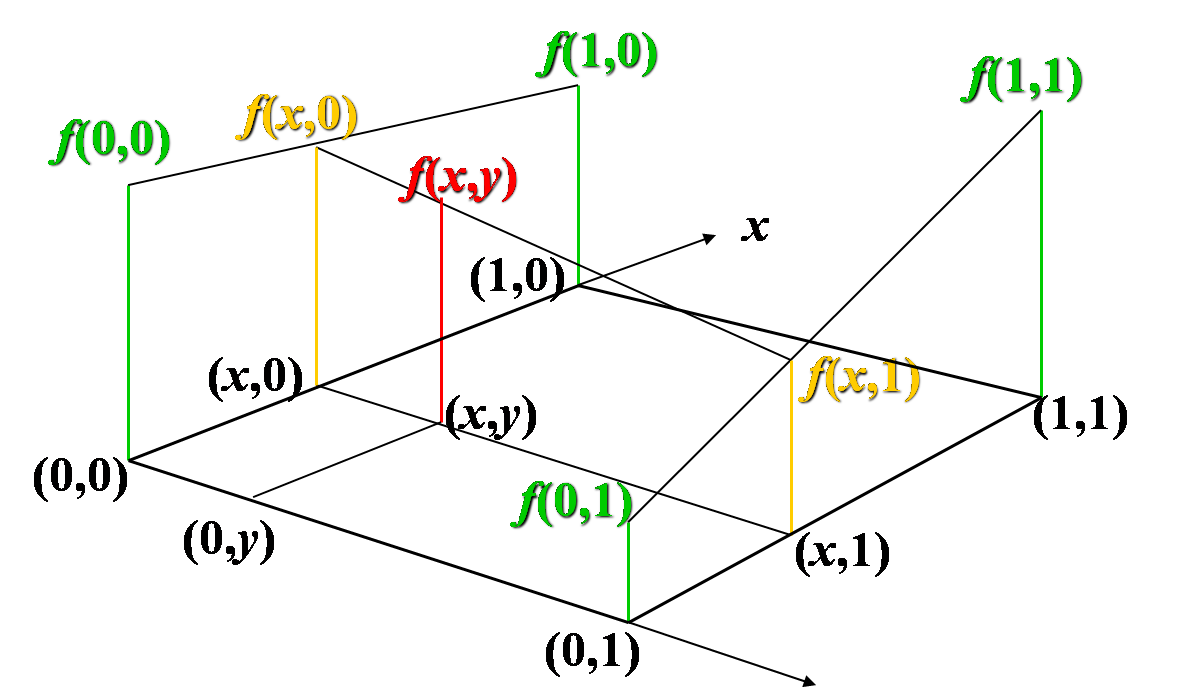# 前想映射后向映射的双线性插值算法

(xy)=(f(x,y)g(x,y))I(x,y)=I(x,y)=I(f(x,y),g(x,y))(1)
(xy) ( x ′ y ′ )
=
(f(x,y)g(x,y)) ( f ( x , y ) g ( x , y ) )
\\ I(x,y)=I'(x',y')=I'(f(x,y),g(x,y)) \tag 1
(xy)=(f1(x,y)g1(x,y))I(x,y)=I(x,y)=I(f1(x,y),g1(x,y))(2)
(xy) ( x y )
=
(f1(x,y)g1(x,y)) ( f − 1 ( x ′ , y ′ ) g − 1 ( x ′ , y ′ ) )
\\ I'(x',y')=I(x,y)=I(f^{-1}(x',y'),g^{-1}(x',y')) \tag 2f(x,y)=(1x)(1y)f(0,0)+(1x)yf(0,1)+x(1y)f(1,0)+xyf(1,1)
f(x,y)=(1-x)*(1-y)*f(0,0)+(1-x)*y*f(0,1)+x*(1-y)*f(1,0)+x*y*f(1,1)

f(0,0)=(1x)(1y)f(x,y)f(0,1)=(1x)yf(x,y)f(1,0)=x(1y)f(x,y)f(1,1)=xyf(x,y)
f(0,0)=(1-x)*(1-y)*f(x,y) \\ f(0,1)=(1-x)*y*f(x,y) \\ f(1,0)=x*(1-y)*f(x,y)\\ f(1,1)=x*y*f(x,y)

(xy)=(αββα)(xy)+((1α)x0βy0βx0+(1α)y0)
[Math Processing Error] ( x ′ y ′ ) =
[Math Processing Error] ( α β − β α )
[Math Processing Error] ( x y ) +
[Math Processing Error] ( ( 1 − α ) x 0 − β y 0 β x 0 + ( 1 − α ) y 0 )09-24686803-107977
04-041万+
12-045535
10-125930
04-227万+
10-20709
05-221万+
07-26140
02-124475
11-173448
09-162669
07-25264
05-032万+
08-18147
03-052342
07-24657
03-12844
©️2020 CSDN 皮肤主题: 大白 设计师:CSDN官方博客